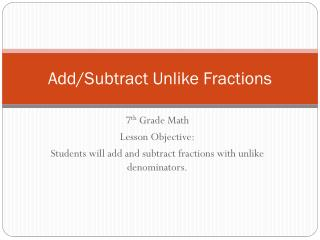DownloadDownload PresentationDownload Presentation- - - - - - - - - - - - - - - - - - - - - - - - - - - E N D - - - - - - - - - - - - - - - - - - - - - - - - - - -
##### Presentation Transcript

1. Add/Subtract Unlike Fractions 7th Grade Math Lesson Objective: Students will add and subtract fractions with unlike denominators.

2. Vocabulary • Unlike Fractions • Fractions with different denominators

3. Steps • Rename the fractions using the least common denominator (LCD) • Add or subtract as with like fractions • If necessary, simplify the sum or difference

4. Add Unlike Fractions 1 • Find ½ + ¼

5. Add Unlike Fractions 2 • Find 9/10 + (-1/2)

6. Add Unlike Fractions - Practice • Add. Write in simplest form.

7. Subtract Unlike Fractions 1 • Find 2/3 – 1/2.

8. Subtract Unlike Fractions 2 • Find 1/2 – (-2/5)

9. Subtract Unlike Fractions - Practice • Subtract. Write in simplest form.

10. Real World Example • Cassandra cuts 5/16 inch off the top of a photo and 3/8 inch off the bottom. How much shorter is the total height of the photo now?

11. Real World Example - Practice • A bucket was 7/8 fill with soapy water. After washing the car, the bucket was only ¼ full. What part of the water was used?